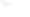# B Tech 3rd Semester 2010-11

Note : (1) Attempt ali the questions.

(2) All questions carry equal marks.

1.Attempt any two parts of the following :

(a) (i) Convert the following numbers as indicated :

(A) (62-7), = ( ),6 = ( )2

(B)  (BC64)|S = ( )|Q = ( ),

(C)  (111011)2 = ( )5

(ii) Represent the unsigned decimal number 965 and 672 in BCD and then show the steps necessary to find their sum.

(b)   (i) Minimize the given Boolean function using K-Map

and implement the simplified function using NAND gates only F(A, B, C, D) = lm(0, 1, 2, 9, 11, 15) + d(8, 10, 14).

(ii) (A) Express the Boolean function : F-AB+AC + AD in a sum of minterms form. (B) Implement two input XOR gate using NOR gates only.

(c)   Consider a (7, 4) cyclic code. The generator polynomial for this code is given as g(x) = 1 + x + x3. Find all the code words of this code.

2. Attempt any four parts of the following :

(a)    Implement the function : F(A, B,C)-ABC + ABC + ABC + ABC using a 4 : 1 multiplexer.

(b) Implement the full subtractor using a 1 : 8 demultiplexer.

(c)   Design a single bit magnitude comparator.

(d)    Design an Excess-3 to BCD code converter.

(e)    Design an octal to binary encoder.

3. Attempt any four parts of the following :

(a)   Explain how SR-FF is converted into D-FF.

(b)  Explain the working of the master slave JK flip-flop.

(c)   Design modulo 3-counter using S-R flip-flop.

(d)   Design a circuit that implements the state diagrams of figure 1.Figure 1

(e) Design a 4 bit serial in-serial out shift register using JK flip-flop.

(f) Design a shift register counter to generate a sequence length of 5 having self-start feature.

4. Attempt any four parts of the following :

(a) Design a combinational circuit using a ROM that accepts a 3 bit number and generates an output binary number equal to the square of the input number.

(b) Implement the following functions using 3-input, 3 product terms and 2 output PLA :

F, =AB + AC

f2=ac + bc.

(c)   It is required to obtain a memory s\$ for a certain application. Given that me are 1K * 8. Obtain the desired system.

(d) Draw and explain the ASM chart for binary multiplexer.

(e)  Explain the basic elements of the ASM chart. How does it differ from conventional flow chart ?

(f)  Distinguish between SRAM and DRAM. Also draw static RAM cell.

5. Attempt any two parts of the following :

(a)  Write short notes on :

(i)   Fundamental mode asynchronous sequential circuit

(ii)  Pulse mode asynchronous sequential circuit.

(b)  (i) What are critical race and non-critical race ? How can they be avoided ? Is race-around condition an example of race ?

(ii)      Design a JK-FF asynchronous sequential circuit that has two inputs and single output. The circuit is required to give an output equal to 1 if and only if the same input variable changes two or more times consecutively.

(c) Suppose the circuit of Figure 2 is operating in fundamental mode. Analyse the circuit by forming the :

(i)    Flow table,

(ii)  Transition flow diagram, and

(iii) Transition flow table if exists.### 2 thoughts on “UPTU Previous Year Exam Papers Digital Logic Design B Tech 3rd Semester 2010-11”

1.Plzz provide me question paper of digital logic design of 2011-2015

2.